### CONVERT Function – Convert Units in Excel, VBA, Google Sheets

This Tutorial demonstrates how to use the Excel CONVERT Function in Excel to convert a number from one unit to another.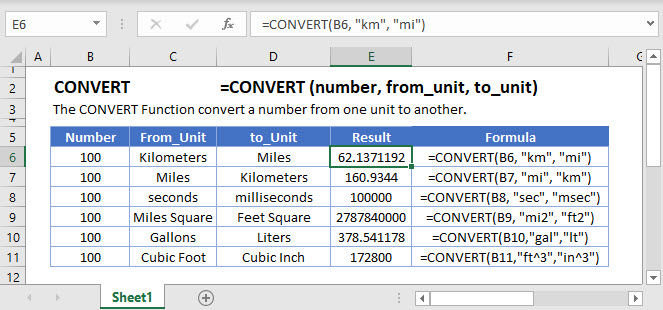## CONVERT Function Overview

The CONVERT Function Converts a number from one measurement unit to another.

To use the CONVERT Excel Worksheet Function, select a cell and type: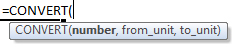(Notice how the formula inputs appear)

## CONVERT function Syntax and inputs:

number – Number of units.

from_unit – The original measurement units.

to_unit – The converted measurement units

AutoMacro - VBA Code Generator

## CONVERT Function

The CONVERT Function converts a number from a measurement system to another. Say, you want to convert miles into kilometers.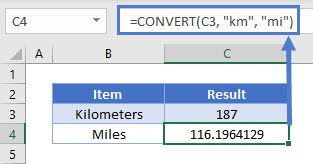## CONVERT Function – Prefixes

The CONVERT Function can also convert from one prefix to another.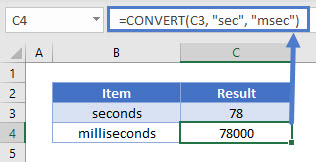Note: The prefix will proceed the unit in the formula.

## CONVERT Function – Binary Prefixes

The CONVERT Function can be used for binary prefixes as well.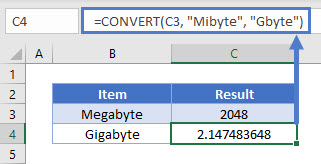## CONVERT Function – Area/Volume

The CONVERT Function can also convert compound units like meter squared or cubic feet.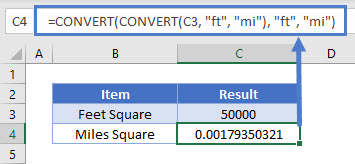Alternatively, the units can be directly entered in the formula.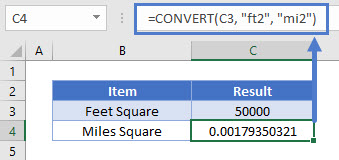## CONVERT Function – Incorrect Data Type

The CONVERT Function will return an error if the input data type is not the same.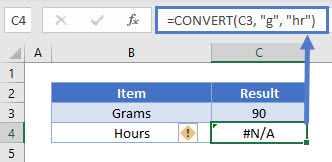The CONVERT Function works exactly the same in Google Sheets as in Excel: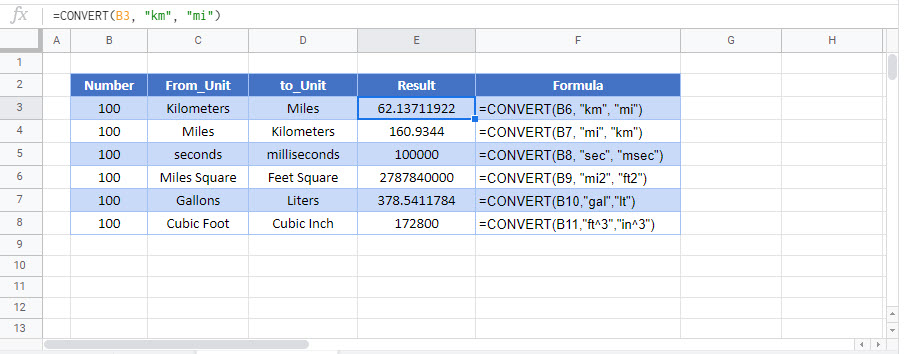Simply enter the number of units, the previous units of measurement, and the desired units of measurement.

## CONVERT Examples in VBA

You can also use the CONVERT function in VBA. Type:
`application.worksheetfunction.convert(number,from_unit,to_unit)`
For the function arguments (number, etc.), you can either enter them directly into the function, or define variables to use instead.